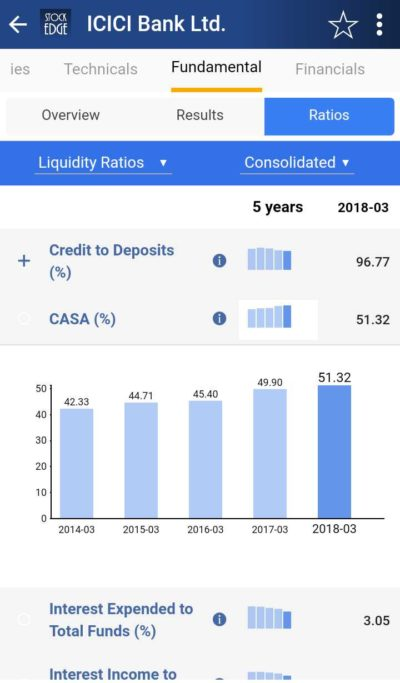StockEdge Blog

## CASA Ratio (%)

All of us have heard about savings account and current account but none of us knows what it actually is. Well, a savings account is opened by an individual and current account by a company. Savings account receives interest while current account receives none.

CASA ratio stands for total deposits in relation to current and savings account. CASA ratio of a bank is the ratio of deposits in current and savings accounts compared to its total deposits. Banks do not give any interest on current account deposits and the interest on a savings account is usually very low between 3-4%.

### Importance

The CASA ratio indicates how much of a bank’s total deposits are in current and savings accounts. A higher ratio means a larger portion of a bank’s total deposits are in current and savings accounts, thus indicating, lower cost of funds. Which means the higher the deposits in both of these accounts the lower will be the cost of managing the funds. Banks pay interest at 3-4% in savings which exceeds the lending rate thus decreasing the management cost. For instance, most banks lend at over 10%, whereas, the rate of interest that they pay on savings deposit is just 3.5%.

### Impact

If a large part of a bank’s deposits comes from these funds, it means that the bank is getting those funds at a relative lower cost. It is generally understood that a higher CASA ratio leads to higher net interest margin. NIIM is used as one of the metrics to assess the profitability of a bank. Now, net interest margin means the difference between interest earned and interest paid. The banks generate higher margins by lending out of CASA deposits. Therefore, higher the CASA ratio, better the net interest margin, which means better operating efficiency of the bank.

### Formula

CASA Ratio (%) = CASA Deposits X 100

Total Deposits

### Explanation

Suppose a bank ABC has total deposits as Rs. 50,000 crore and savings account deposits is Rs. 15000 crores and current account deposits is Rs. 8,000 crores.

CASA Ratio (%) = 15000 + 8000 X 100 = 46%

50,000

Thus, the CASA ratio of the bank is 46% which means 46% of total deposits are contributed by low-cost CASA deposits.CASA

### Stockedge App

We don’t have to calculate CASA ratio (%) with the stockedge app. StockEdge gives us the CASA ratio of the last five years of any banks listed in the stock exchange. We can look and compare CASA ratio (%) of any banks and filter out stocks accordingly.

Suppose we want to look at CASA ratio of ICICI Bank Ltd.for last 5 years, then in the Fundamental tab of ICICI Bank Ltd., we will get Ratios tab.  Then in the Ratios tab click on the Liquidity Ratios, we will get CASA ratio (%) of ICICI Bank Ltd.

### Bottomline

CASA (%) of any bank is an important financial component to look at when analyzing a Bank. It tells us about profitability and operating efficiency of a bank. It can be compared on time basis and with other banks to understand the strength of the banks. With a click of a button, you can see the bank’sCASA ratio (%) for five years.